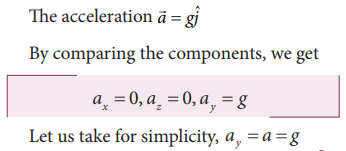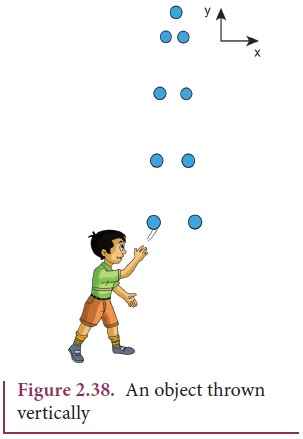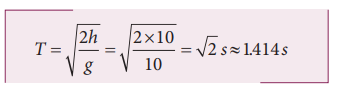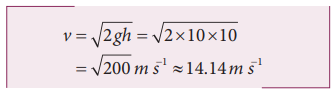Home | | Physics 11th std | Equations of motion under gravity

# Equations of motion under gravity

Case (1): A body falling from a height h Case (ii): A body thrown vertically upwards

Equations of motion under gravity

A practical example of a straight line motion with constant acceleration is the motion of an object near the surface of the Earth. We know that near the surface of the Earth, the acceleration due to gravity ‘g’ is constant. All straight line motions under this acceleration can be well understood using the kinematic equations given earlier.

Case (1): A body falling from a height hConsider an object of mass m falling from a height h. Assume there is no air resistance. For convenience, let us choose the downward direction as positive y-axis as shown in the Figure 2.37. The object experiences acceleration ‘g’ due to gravity which is constant near the surface of the Earth. We can use kinematic equations to explain its motion. We haveIf the particle is thrown with initial velocity ‘u’ downward which is in negative y axis, then velocity and position at of the particle any time t is given byThe square of the speed of the particle when it is at a distance y from the hill-top, isSuppose the particle starts from rest.

Then u = 0

Then the velocity v, the position of the particle and v2 at any time t are given by (for a point y from the hill-top)The time (t = T) taken by the particle to reach the ground (for which y = h), is given by using equation (2.15),The equation (2.18) implies that greater the height(h), particle takes more time(T) to reach the ground. For lesser height(h), it takes lesser time to reach the ground.

The speed of the particle when it reaches the ground (y = h) can be found using equation (2.16), we getThe above equation implies that the body falling from greater height(h) will have higher velocity when it reaches the ground.

The motion of a body falling towards the Earth from a small altitude (h << R), purely under the force of gravity is called free fall. (Here R is radius of the Earth )

Case (ii): A body thrown vertically upwards

Consider an object of mass m thrown vertically upwards with an initial velocity u. Let us neglect the air friction. In this case we choose the vertical direction as positive y axis as shown in the Figure 2.38, then the acceleration a =-g (neglect air friction) and g points towards the negative y axis. The kinematic equations for this motion are,The velocity and position of the object at any time t are,The velocity of the object at any position y (from the point where the object is thrown) is## Solved Example Problems for motion under gravity: Case (1): A body falling from a height h

Example 2.34

An iron ball and a feather are both falling from a height of 10 m.

a) What are the time taken by the iron ball and feather to reach the ground?

b) What are the velocities of iron ball and feather when they reach the ground?

(Ignore air resistance and take g = 10 m s-2)

### Solution

Since kinematic equations are independent of mass of the object, according to equation (2.8) the time taken by both iron ball and feather to reach the ground are the same. This is given byThus, both feather and iron ball reach ground at the same time.

By following equation (2.19) both iron ball and feather reach the Earth with the same speed. It is given by### Example 2.35

Is it possible to measure the depth of a well using kinematic equations?Consider a well without water, of some depth d. Take a small object (for example lemon) and a stopwatch. When you drop the lemon, start the stop watch. As soon as the lemon touches the bottom of the well, stop the watch. Note the time taken by the lemon to reach the bottom and denote the time as t.

Since the initial velocity of lemon = 0 and the acceleration due to gravity g is constant over the well, we can use the equations of motion for constant acceleration.Since u = 0, s = da = g (Since we choose the y axis downwards), ThenSubstituting g = 9.8 m s-2 we get the depth of the well.To estimate the error in our calculation we can use another method to measure the depth of the well. Take a long rope and hang the rope inside the well till it touches the bottom. Measure the length of the rope which is the correct depth of the well (dcorrect ). ThenWhat would be the reason for an error, if any?

Repeat  the  experiment  for  different masses and compare the result with dcorrect every time.

## Solved Example Problems for motion under gravity: Case (2): A body thrown vertically upwards

### Example 2.36

A train was moving at the rate of 54 km h-1 when brakes were applied. It came to rest within a distance of 225 m. Calculate the retardation produced in the train.

### Solution

The final velocity of the particle v = 0

The initial velocity of the particleRetardation is always against the velocity of the particle.Tags : with Solved Example Problems , 11th Physics : UNIT 2 : Kinematics
Study Material, Lecturing Notes, Assignment, Reference, Wiki description explanation, brief detail
11th Physics : UNIT 2 : Kinematics : Equations of motion under gravity | with Solved Example Problems